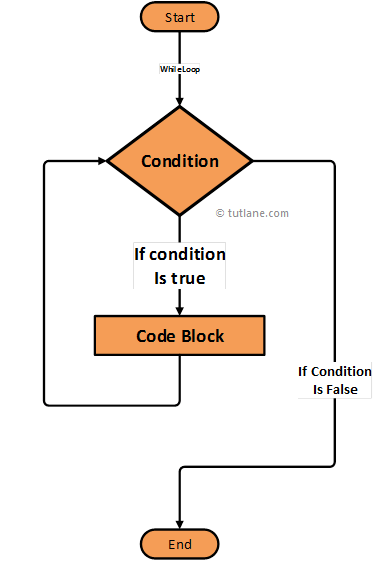# Swift While Loop

In swift, while loop is used to execute a set of statements continuously until the defined condition is TRUE.

Generally in swift, if we use for loop, we will clearly know that how many times the given statements will execute repeatedly but in while loop we don’t know how many times the given statements will execute repeatedly until the defined condition fails (FALSE).

In swift, while loop is useful whenever we need to execute given statements repeatedly till the defined condition is TRUE but not aware of how many times the statements will execute repeatedly.

In swift, while loop will evaluate the condition before starting of the loop. Now we will see the functionality of while loop in a swift programming language using an algorithm diagram.

## Swift While Loop Flow Diagram

Following is the swift while loop statement flow diagram to represent how the functionality of while loop will work in a swift programming language.If you observe above swift while loop flow diagram first it will check the condition and start execution of statements until the defined condition fails.

## Syntax of Swift While Loop

Following is the syntax of defining while loop in a swift programming language.

while condition {

}

If you observe above swift while loop syntax first it will check the condition and execute the statements until the defined condition fails.

Now we will see how to use while loop in swift programming language with examples.

## Swift While Loop Example

Following is the simple example using while loop in swift to execute statements repeatedly till the condition TRUE.

var i = 1

while i < 15 {

print(i)

i = i + 2

}

If you observe above while loop example first it will check whether the variable “i” value less than 15 or not. In case if it less than 15 then it will print the value and continue the loop till the defined condition fails (FALSE).

When we execute the above program in swift playground we will get a result like as shown below.

1

3

5

7

9

11

13

This is how we can use while loop in a swift programming language to execute set of statements repeatedly till the defined condition fails based on our requirements.